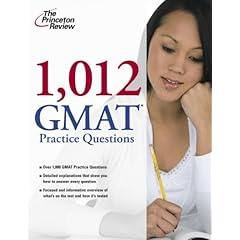# Princeton Review 1,012 GMAT Practice Questions Book Review

- Jun 17, 00:49 AM Comments##### Princeton Review 1,012 GMAT Practice Questions (Look up details on Amazon)

The book indeed contains 1,012 questions - counted myself :). It starts with a diagnostic test (37q and 41v questions). There are a total of 55 different "drills" targeting various interesting and often neglected areas. The format for Math is not just standard multiple choice, but actually 50% of the quant questions are Data Sufficiency, so one can practice both questions types - PS and DS.

Book Contents and Question Split:

• Quant:
• Arithmetic (terminology, percents, multiples, fractions, ratios, factors, decimals) - 70 questions
• Algebra (equations and inequalities, plugging in, pita, quadratics, must be true, Yes/No DS, roots, interest, functions, powers) - 163 questions
• Statistics, Probability, and Combinations (averages, median, mode, range, stdev, rate, factorials, probability, permutations and combinations, groups) - 117 questions
• Geometry (lines and angles, triangles, quadraliterlas, circles, 3D figures, coordinate geometry, inscribed figures and shaded regions) - 100 questions
• Quant Subtotal: 450
• Verbal:
• RC - 30 passages and 150 questions (5 questions per passage)
• SC (subject/verb agreement, tenses, pronouns, parallel, modifiers, idioms, mixed) - 175 questions
• CR (weaken, strengthen, assumption, inference, evaluate, ID reasoning, resolve/explain, mixed) - 159 questionss
• Verbal Subtotal: 484
• Total: 934 + diagnostic test (78) = 1,012

I am giving Kudos for these sections: factorials, probability, combinations, groups, Yes/No DS, assumption, and parallel structure questions. These areas are often neglected by test prep companies and I am thrilled to see a guide that actually addresses these.

Issues I have with this book:
- Random House used cheap paper - as always
- RC section is structured by topic (humanities, science, etc rather than by type of questions)
- Verbal questions are a bit off (esp. CR) but that's to be expected
- Could use more 700-level questions but there are a few in each section

See my other reviews for overview of the latest GMAT Books: